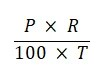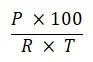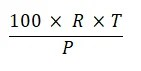Breaking News

# Financial Mathematics – Simple Interest MCQs with Answers

Financial Mathematics – Simple Interest MCQs with Answers

Correct! Wrong!

Correct! Wrong!

Correct! Wrong!

Correct! Wrong!

Correct! Wrong!

Correct! Wrong!

Correct! Wrong!

Correct! Wrong!

#### 9. The formula for simple interest is:Correct! Wrong!

Correct! Wrong!

Correct! Wrong!

Correct! Wrong!

#### What is the rate of simple interest for the first 4 years if the sum of Rs. 360 becomes Rs. 540 in 9 years and the rate of interest for the last 5 years is 6%?

Correct! Wrong!

Original sum = Rs 360 Total interest in 9 years = 540 - 360 = 180 Interest in last 5 years = 5 x 6 = 30% = 30/100 x 360 = Rs 108 => Interest in first four years : 180 - 108 = Rs 72 Interest per annum = 72/4 = 18 Rate of interest for first four years = 18/360 x 100 = 5% Check : Interest for four years = 4 x 5 = 20% Interest for next five years = 5 x 6 = 30% Total interest = 20% + 30% = 50% Now 50% of 360 = Rs 180

Correct! Wrong!

Correct! Wrong!

## Entrepreneurship MCQs with Answers Part # 2

Entrepreneurship MCQs with Answers Part 2 Questions 51 to 70 Questions 71 to 100 Click …